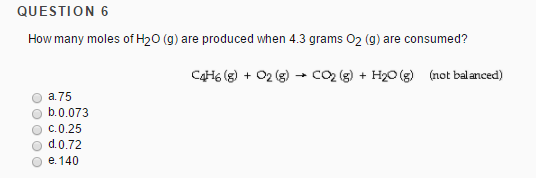# Problem: How many moles of H2O(g) are produced when 4.3 grams O2(g) are consumed? C4H6(g) + O2(g) → CO2(g) + H2O(g) (not balanced)a. 75 b. 0.073c. 0.25d. 0.72e. 140

###### FREE Expert Solution
93% (335 ratings)###### Problem Details

How many moles of H2O(g) are produced when 4.3 grams O2(g) are consumed? C4H6(g) + O2(g) → CO2(g) + H2O(g) (not balanced)

a. 75

b. 0.073

c. 0.25

d. 0.72

e. 140